Updated by becca21-34-ba on Mar 24, 2016
REPORT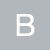becca21-34-ba
Owner
10 items   1 followers   0 votes   4 views

1Description- This website focuses on explaining how to solve the quadratic equation.
Rationale- I chose this resource because it seems easy for the students to navigate and it has very specific and useful information for them to use about the quadratic equation.

2

## What Is a Quadratic Equation? - free Mathematics lessons and testsDescription- This website explains the basics of the quadratic equation and the quadratic formula. It also gives the graph.
Rationale- I chose this website because it has very specific and useful information on the quadratic equation. It is also easy for the students to navigate.

3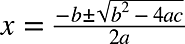Description- This picture shows the quadratic formula.
Rationale- This picture is a visual aid that I can use to help the students remember the quadratic formula.

4

## Quadratic Formula Pop Goes the Weasel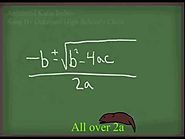Description- This video is a song for the quadratic formula that goes to the tune of pop goes the weasel.
Rationale- This is very specific to the quadratic formula and will help the students remember because it is to a catchy tune.

5

## Quadratic Equation Real World ApplicationDescription- This picture shows that there are real world applications to using and solving quadratic equations.
Rationale- This is a very visual picture and helps the students understand that there are things that they can use the quadratic equation for in the real world.

6Rationale- This website is visually appealing and has very useful information for the students. It also has many links that can help them with specifics that they might not understand.

7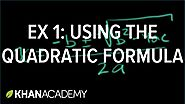Description- This video provides a visual element for students so they can walk through the use of the quadratic formula with the video.
Rationale- This video is very visual and will help the students that learn better through seeing an example being worked out. Khan Academy is a very reliable resource to use.

8Description- This website is explaining roots of the quadratic equation.
Rationale- This is very helpful for the students to fully understand why they have to solve the equation and what it means on the graph.

9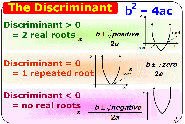Description- This picture is a visual representation of the use of a discriminant to determine how many real roots are on the graph.
Rationale- This is very useful because it helps the students determine how many real roots are on the graph. It is also very visually appealing and can be easy to understand.

10

## Quadratic Functions and Parabolas in the Real World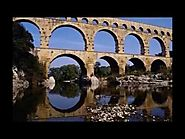Description- This video gives real world examples of when quadratic functions and parabolas are used.
Rationale- This is very visual for the students and could possibly peak their interests in the topic more because of the real world applications.